## Saturday, October 21, 2006

### Bernoulli Numbers

The Bernoulli numbers were first identified by Jakob Bernoulli in Ars Conjectandi, a work that was published after his death. The Bernoulli numbers turn up again and again in the history of mathematics. In today's blog, I will focus on the original problem that Jakob Bernoulli was attempting to solve. In a future blog, I will show how they were used by Ernst Kummer as a criteria for determining if a prime is regular.

Interestingly, Bernoulli numbers may have been discovered earlier by Seki Kowa and were rediscovered independently by a 17-year-old Srinivasa Ramanujan.

Bernoulli was analyzing formulas for the sums of powers, that have the following form:

Sm(n) = ∑ (i=1, n) im

He looked at, for example:

S0(n) = n

S1(n) = (1/2)n2 - (1/2)n

S2(n) = (1/3)n3 - (1/2)n2 + (1/6)n

S3(n) = (1/4)n4 - (1/2)n3 + (1/4)n2

S4(n) = (1/5)n5 - (1/2)n4 + (1/3)n3 - (1/30)n

S5(n) = (1/6)n6 - (1/2)n5 + (5/12)n4 - (1/12)n2

S6(n) = (1/7)n7 - (1/2)n6 + (1/2)n5 - (1/6)n3 + (1/42)n.

S7(n) = (1/8)n8 - (1/2)n7 + (7/12)n6 - (7/24)n4 + (1/2)n2

S8(n) = (1/9)n9 - (1/2)n8 + (2/3)n7 - (7/15)n5 + (2/9)n3 - (1/30)n

S9(n) = (1/10)n10 - (1/2)n9 + (3/4)n8 - (7/10)n6 + (1/2)n4 - (3/20)n2

S10(n) = (1/11)n11 - (1/2)n10 + (5/6)n9 - n7 + n5 - (1/2)n3 + (5/66)n.

By looking at these formulas, he noticed a pattern and in analyzing this pattern, he came up with what were termed Bernoulli numbers by Abraham de Moivre.

Well, for each Sm(n), we can see that:

1) the first term is 1/(m+1)*nm+1

2) the second term, when it exists, is (-1/2)nm

3) the third term, when it exists, is (m/12)*nm-1

4) the fourth term, when it exists, is (-m)(m-1)(m-2)/720*nm-3

Bernoulli had discovered that if he defined a sequence of the numbers, he got a simple formula for defining the sum of powers.

The formula he discovered was the following:In the above formula, Bk refers to the sequence of Bernoulli numbers where Bernoulli numbers are defined in the following way:

Definition 1: Bernoulli Numbers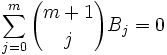with B0 = 1.

As a convention, I will refer to Bernoulli numbers as Bi.

So, to figure out, B1, we have: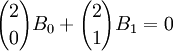This gives us:

2*B0 + 2*B1 = 0

B1 = (1/2)[-2*B0] = (-1/2)(1) = (-1/2)

We determine B2 based on solving: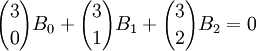In this way, we find that:

B0 = 1
B1 = -(1/2)
B2 = (1/6)
B3 = 0
B4 = -(1/30)
B5 = 0
B6 = (1/42)
B7 = 0
B8 = -(1/30)
B9 = 0
B10 = (5/66)
B11 = 0
B12 = -(691/2730)

Bernoulli wrote that he was able to use his formula to compute S10(1000) in less than 10 minutes (see Smith, A Source Book in Mathematics, below).

Using his formula, this comes down to: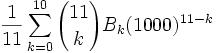Evaluating this gives us:

91,409,924,241,424,243,424,241,924,242,500

I will now show a proof for Bernoulli's formula for sums. This is taken straight from Graham et al. Concrete Mathematics.

Definition 2: Sm(n)

Let Sm(n) = 0m + 1m + ... + (n-1)m

I will use this shorthand in the lemma and theorem below.

Postulate 1: 00 = 1

(This is taken from Graham et al.)

Graham et al argue in Concrete Mathematics that if we let 00 = 1, it provides a more elegant notation for the Binomial Theorem. Their argument is that the Binomial Theorem is very important while 00 is almost irrelevant. (see Concrete Mathematics, page 162).

Lemma 1: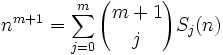Proof:

(1) Sm+1(n) + nm+1 =

= ∑(k=0,n-1) km+1 + nm+1 =

= ∑(k=0,n) km+1 =

= 0m+1 + ∑(k=1,n) km+1 = ∑(k=1,n) km+1 =

= ∑ (k=0,n-1) (k+1)m+1

(2) Using the Binomial Theorem, we get: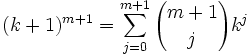(3) Putting this together with step #1 gives us: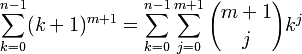This gives us: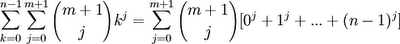(4) Since Sj(n) = oj + 1j + ... + (n-1)j, we have shown that: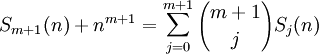(5) Now, since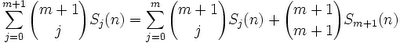The conclusion follows from simple subtraction since [(m+1)!]/[(m+1)!(0)!] = 1.

QED

Theorem: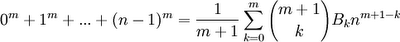Proof:

(1) We can see that this is true for m=0 since:

S0 = n = (1/[1+1])∑ (k=0,0) ([0+1]!/k![1-k]!)Bkn0+1-k =

= (1)*(1!/[0!][1!])B0n1 = (1)*(1)*(1)*n = n [Since B0 = 1, see above]

(2) Let's assume that this is true up to m-1.

(3) Let Tm(n) =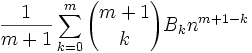(4) Let Δ = Sm(n) - Tm(n)

(5) Using Lemma 1 above, we know that:(6) Using our definition of Δ, we can also see that:(7) The goal in this proof will be to show that Δ = 0.

(8) Adding the definition of Tj(n) [see step#3] gives us: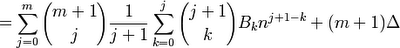I also simplified [(m+1)!/(m!)(1!)] to (m+1).

(9) We can move all values that are independent of k before the ∑ (k=0,j):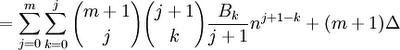(10) Since we can change the order of the terms of a finite sum and still get the same result, we can switch each value of k with (j-k) to get: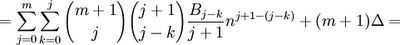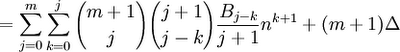(11) Now, since (j+1) - (j-k) = k+1, we have: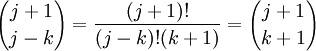(12) Putting this result in step #11 gives us: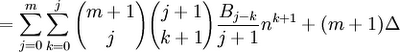(13) We can see that in step #12, 0 ≤ k ≤ j ≤ m. The sum is in fact all combinations of j,k where this is the case. This means that we can rearrange the sum to the following: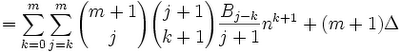(14) Since nk+1 is independent of j, we can move it over to get the following: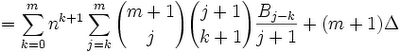(15) Since,we can also rearrange the result in step #14 to give us: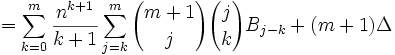(16) Since: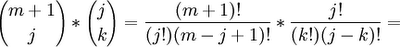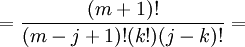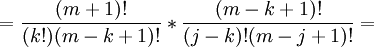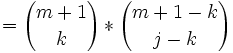We can rearrange terms to get: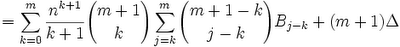(17) Since: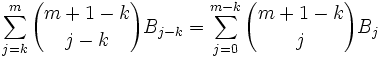We can rearrange terms to get:(18) Now, we know from the definition of Bernoulli numbers (see Definition 1 above) thatin all cases except where m = 0. This occurs only when k = m.

This allows us to simplify the result in step #17 to the following: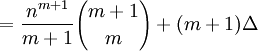(19) Now since [(m+1)!/(m!)(1!)] = m+1, we get:

= nm+1 + (m+1)Δ

(20) But in step #5, we started out with nm+1 so we are left with:

nm+1 = nm+1 + (m+1)Δ.

(21) The only way that this is true is if Δ = 0.

QED

In another blog, I show how z/[ez - 1] can be used as a generating function for the Bernoulli numbers.

References